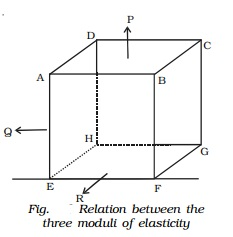Home | | Physics | Relation between the three moduli of elasticity

# Relation between the three moduli of elasticitySuppose three stresses P, Q and R act perpendicular to the three facesABCD, ADHE and ABFE of a cube of unit volume (Fig.). Each of these stresses will produce an extension in its own direction and a compression along the other two perpendicular directions.

Relation between the three moduli of elasticity

Suppose three stresses P, Q and R act perpendicular to the three facesABCD, ADHE and ABFE of a cube of unit volume (Fig.). Each of these stresses will produce an extension in its own direction and a compression along the other two perpendicular directions. If λ is the extension per unit stress, then the elongation along the direction of P will be λP. If � is the contraction per unit stress, then the contraction along the direction of P due to the other two stresses will be �Q and �R.The net change in dimension along the direction of P due to all the stresses is e = λP - �Q - �R.

Similarly the net change in dimension along the direction of Q is f = λQ - �P - �R and the net change in dimension along the direction of R is g = λR - �P - �Q.

Case (i)

If only P acts and Q = R = 0 then it is a case of longitudinal stress.

Linear strain = e = λP

Young's modulus q =linear stress /linear strain = P / λP

q= 1/λ

or

λ = 1/q       �����....(1)

Case (ii)

If R = O and P = - Q, then the change in dimension along P is e = λP - � (-P)

(i.e) e = (λ + �) P

Angle of shear θ = 2e* = 2 (λ + �) P

Rigidity modulus

n = P/θ

= P/ 2(λ+ �)P   �����..(2)

Case (iii)

If P = Q = R, the increase in volume is = e + f + g

= 3 e = 3 (λ − 2�) P (since e = f = g)

Bulk strain = 3(λ−2�) P

Bulk modulus k = P / 3(λ - 2�)P

Or   (λ − 2�)= 1/3k            �����(3)

From (2), 2(λ + �) = 1/n

2λ + 2� = 1/n

From (3), (λ − 2�) = 1/3k

3λ = 1/n + 1/3k

λ = 1/3n + 1/9k

From (1),

1/q = 1/3n + 1/9k

9/q = 3/n + 1/k

This is the relation between the three moduli of elasticity.

Study Material, Lecturing Notes, Assignment, Reference, Wiki description explanation, brief detail
11th 12th std standard Class Physics sciense Higher secondary school College Notes : Relation between the three moduli of elasticity |